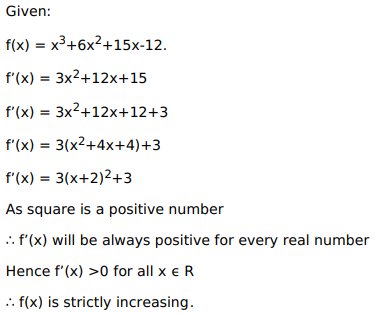# Solve this following

Question:

Mark $(\sqrt{)}$ against the correct answer in the following:

The function $f(x)=x^{3}+6 x^{2}+15 x-12$ is

A. strictly decreasing on $R$

B. strictly increasing on R

C. increasing in $(-\infty, 2)$ and decreasing in $(2, \infty)$

D. none of these

Solution: